Lei, J., Wang, S., Lei, D., and Liu, Z. (2022). "Measurement and calibration of discrete element simulation parameters of crushed sugarcane tail leaves," BioResources 17(4), 5984-5998.

#### Abstract

Discrete element simulation parameters of the tail stem and tail leaves of crushed sugarcane tail leaves (STL) were calibrated by a combination of physical experiments and simulation optimization design. First, the values or ranges of the basic physical parameters and contact parameters of crushed STL were measured using physical tests, and the results were used as the basis for the selection of the simulation parameters. Plackett-Burman testing was applied for the significance screening of the initial parameters. Then, the error values and significant parameters of stacking angle for the second-order regression models were obtained using the steepest ascent experiment and the Box-Behnken optimization test. An analysis of variance (ANOVA) was also performed. Finally, using 37.52° stacking angle of physical test as the validation target, the optimal combination of parameters was obtained: coefficient of static friction (COSF) for tail stem-tail stem of 0.45, COSF for tail leaf-tail leaf of 0.38, coefficient of rolling friction (CORF) for tail stem-tail stem of 0.14, and CORF for tail stem-tail leaf of 0.12. The error of stacking angle obtained from the simulation and the physical tests was 0.976%, which verifies the reliability of the optimal parameters.

Measurement and Calibration of Discrete Element Simulation Parameters of Crushed Sugarcane Tail Leaves

Junle Lei,* Shuaiwei Wang, Dingyuan Lei, and Zhaochong Liu

Discrete element simulation parameters of the tail stem and tail leaves of crushed sugarcane tail leaves (STL) were calibrated by a combination of physical experiments and simulation optimization design. First, the values or ranges of the basic physical parameters and contact parameters of crushed STL were measured using physical tests, and the results were used as the basis for the selection of the simulation parameters. Plackett-Burman testing was applied for the significance screening of the initial parameters. Then, the error values and significant parameters of stacking angle for the second-order regression models were obtained using the steepest ascent experiment and the Box-Behnken optimization test. An analysis of variance (ANOVA) was also performed. Finally, using 37.52° stacking angle of physical test as the validation target, the optimal combination of parameters was obtained: coefficient of static friction (COSF) for tail stem-tail stem of 0.45, COSF for tail leaf-tail leaf of 0.38, coefficient of rolling friction (CORF) for tail stem-tail stem of 0.14, and CORF for tail stem-tail leaf of 0.12. The error of stacking angle obtained from the simulation and the physical tests was 0.976%, which verifies the reliability of the optimal parameters.

DOI: 10.15376/biores.17.4.5984-5998

Keywords: Sugarcane tail leaf; Discrete element simulation; Stacking angle; Parameter calibration

Contact information: College of Mechanical and Control Engineering, Guilin University of Technology, Guilin, 541004, P. R. China; *Corresponding author: 40911409@qq.com

INTRODUCTION

Sugarcane is an important sugar crop in the world and is widely grown in Guangxi and Yunnan, China (Li et al. 2015; Fan et al. 2020). Sugarcane tail leaves (STL) are the two or three tender nodes at the top of sugarcane and their accompanying whole leaves (mainly including both tail stems and tail leaves) that remain after harvesting, accounting for approximately 20% of the total weight of sugarcane (Junqueira Franco et al. 2009; Zhou et al. 2019). Both cheap and rich in nutrients, STL are excellent silage resources. After being crushed by harvesting machinery, it can meet the conditions of silage and realize storage, which can solve many problems, such as the shortage of green fodder for cattle and sheep in winter and spring seasons, and thus mechanized harvest of STL has important economic and social values (Neto et al. 2014; Pereira et al. 2019). Because of the coarse and hard roots and high water content of STL, problems including uneven crushing, nutrition loss by kneading and extrusion, and easy blockage of conveying channels occur when using traditional forage harvesting machines (Wu et al. 2020; Qiu et al. 2021). The development of a new STL harvesting machine requires structural innovation and optimal design of working parameters for several parts such as crushing, throwing, collecting, and discharging. The application of discrete element method to study the mechanism or interaction between STL and harvesting machine can provide a theoretical basis for the design and innovation of the harvesting machine. The main parameters required for discrete element simulation include: basic physical parameters such as Poisson’s ratio, density, shear modulus of STL; and contact parameters such as coefficient of restitution (COR), coefficient of static friction (COSF), and coefficient of rolling friction (CORF) between STL particles or between particles and machine (Grima and Wypych 2011; Fang et al. 2022; Wang et al. 2022). Obtaining simulation parameters objectively and accurately is a prerequisite for applying the discrete element method to study the mechanism of the interaction between STL and harvesting machine.

In recent years, scholars at home and abroad have conducted a lot of research work to obtain the discrete element simulation parameters (Syed et al. 2017; Ghodki et al. 2019; Qu et al. 2020; Zhang et al. 2020). Huan et al. (2022) used bench testing and simulation testing to obtain the best combination of contact parameters for the discrete element model of king grass stem via the collision and bouncing test and slope sliding test. Wang et al. (2022) discovered that the contact salient parameters of alfalfa straw with different water contents based on the angle of repose are obviously different. Further, the surface energy is an important factor affecting the angle of repose, and the optimal values of the salient parameters under different water contents are determined. Lee and Park (2019) designed four simulated friction tests to measure COSF and CORF between materials or between materials and containers, and verified the accuracy of the measured coefficient of static friction (COF) with the repose angle as the response value. Liu et al. (2020) used a combination of physical test and simulation test to measure the contact parameters of quinoa, and optimized the second-order regression equation with the angle of repose of the physical test as the optimal objective to obtain the best combination of contact parameters.

The STL is a new silage crop with physical properties that differ greatly from existing research crops. However, related studies are few and there is a lack reference to similar materials. Therefore, this paper took crushed STL as the research object, and used a combination of physical tests and simulation to measure the basic physical parameters and contact parameters of crushed STL. Using the stacking angle from the physical test as the response value, the contact parameters that had a considerable effect on the test results were screened by the Plackett-Burman test, and the steepest ascent experiment and Box-Behnken tests were also conducted to calibrate the simulated contact parameters, establish the regression equation, and obtain the optimal combination of simulation parameters. Finally, the accuracy of the discrete element simulation parameters was determined by comparing the measured values of the physical test and the simulation test for the stacking angle of crushed STL to provide a simulation parameter basis for the numerical simulation of STL mechanized harvesting.

EXPERIMENTAL

Determination of Basic Physical Parameters of Crushed Sugarcane Tail Leaves

The STL used were selected from the Gui sugarcane No. 42, a species grown by Guilin branch of Guangxi Academy of Agricultural Sciences, whose moisture content of STL ranged from 65% to 82%. Ten STL were used as samples and crushed, and the crushed STL remained green. The crushed samples mainly contained three parts, including short tail stems, crushed tail leaves, and the impurities, and the average proportion of each part in each sugar cane was measured as 41.3% for short tail stems, 56.1% for crushed tail leaves, and 2.6% for impurities. Because the proportion of impurities was small, after removing them, five crushed STL were randomly taken as samples, and the length and diameter of short tail stems were measured using Vernier calipers with an accuracy of 0.02 mm. The area of the STL sample was measured using square grid paper. The average length and diameter of short tail stems were 5.62 mm and 18.38 mm with coefficients of variation (COV) of 3.42% and 5.94%, respectively. For crushed tails, the average area was 2.12 cm2 and the COV was 8.44%.

The density indicators of STL mainly include density of tail stem and tail leaf. As shown in Fig. 1, the density of tail stem and tail leaf of STL was measured by the overflowing water equals volume method in this paper. Fifty tail stems with a length of 30 mm and fifty tail leaves with a length of 100 mm were randomly selected for measurement, and every ten tail stems and every ten tail leaves were tied into a bundle with a thin thread. The mass of the tail stems and tail leaves were measured with an electronic scale, and the volume of the tail stems and tail leaves was measured using the immersion method. The tail stems and tail leaves were gently placed into a measuring cup filled with pure water, and the top was covered with a flat plate to ensure that all the tail stems and tail leaves were immersed in the water so that the pure water would overflow. After the measuring cup was removed, the volume of the tail stems and tail leaves was obtained by measuring the mass of the overflowing pure water and calculating the volume of the pure water. The test was repeated 5 times. The average density of tail stems and tail leaves of sugarcane were 986.84 kg/m3 and 741.53 kg/m3 and the COV were 6.59% and 7.28%, respectively, as determined by the experiment.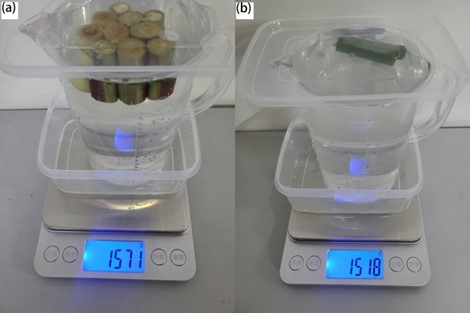Fig. 1. Test picture of density measuring device: (a) Measuring tail stem density; (b) Measuring tail leaf density

Determination of Poisson’s Ratio of Tail Stems

Tail stems with the average diameter of 20 mm were selected from the middle section of the mature STL, and cylindrical samples with a length of 30 mm were made, as shown in Fig. 2(a). The samples were subjected to compression tests using a WDW-10 microcomputer-controlled electronic universal testing machine (Jinan Zhongbiao Instrument Equipment Co., Ltd., Jinan, China), as shown in Fig. 2(b). The loading speed was set to 5 mm/min, and the test was repeated 10 times. The changes of length and diameter before and after the uniaxial compression test were calculated to obtain the tail stems with elastic modulus of 27.26 MPa, the COV was 8.79%, shear modulus was 10.57 MPa, the COV was 7.92%, Poisson’s ratio was 0.29, and the COV was 8.55%.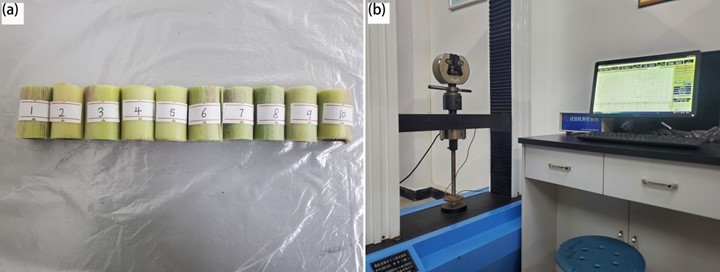Fig. 2. Compression test of STL and tail stems: (a) Compressed samples; (b) Compression testing device

Determination of the Coefficient of Restitution

The test principle is shown in Fig. 3, and the COR of the material was measured using the slanted plate collision method (Mu et al. 2021). The tail stem was released to free fall motion at the height H0 from the collision slope, and without considering the effect of air resistance on the fall of the tail stem, it bounced back at a certain speed after colliding with the collision material (tail leaf, tail stem, or 45 steel) on the collision slope with an inclination of 45° and fell onto the receiving plate. When the relative height between the collision point o and the receiving plate was H1, the horizontal displacement of the tail stem was measured as L1. When the relative height between the collision point o and the receiving plate was H2, the measured horizontal displacement of the tail stem was L2. According to the law of conservation of energy, the COR e between the tail stem and the collision material could be calculated using Eq. 1,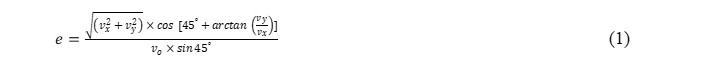where vo is the instantaneous velocity before the collision (mm/s), vx is the horizontal velocity component after the collision (mm/s), and vy is the vertical velocity component after the collision (mm/s).

The following variables vo, vx, and vy are calculated by the following Eqs. 2 to 4: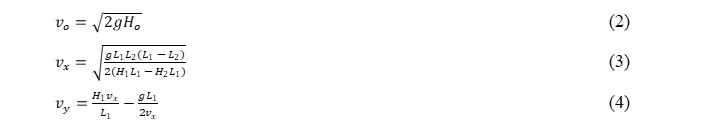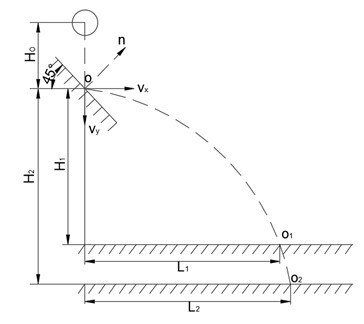Fig. 3. Principle of COR test

A total of 40 tail stems were selected for measurement in this test, 20 in each group, and the height of the receiver plate was changed for two tests. The value of horizontal displacement in x-direction of each group was averaged and recorded, and the COR was calculated by Eqs. 1 to 4, and the measured COR were 0.29, 0.33, and 0.42 for tail stem-tail leaf, tail stem-tail stem, and tail stem-45 steel, with COV of 7.55%, 5.41%, and 5.8%, respectively.

Determination of the Coefficient of Static Friction

The COSF was measured by the slope sliding test (Jia et al. 2021). The tail leaves are tiled and glued on the inclined plane to obtain the test area, and the tail stem with a length of 60 mm was selected to be placed along the length of the inclined plane. The slant plate was lifted counterclockwise slowly and uniformly until the tail stem was observed to start sliding on the slant plate and stop rotating. The inclination angle of the slant plate installed on one side of the measuring instrument was recorded. The formula for calculating the COSF was Eq. 5,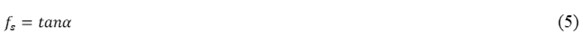where fs is the COSF and α is the inclination angle of the inclined plate (°).

Each group of tests was repeated 10 times to obtain the range of COSF between the tail stem and tail leaf. The same method was used to measure the range of COSF between the tail stem, tail leaf and 45 steel, respectively, and the results are shown in Table 1.

Table 1. Results of COSF Measurement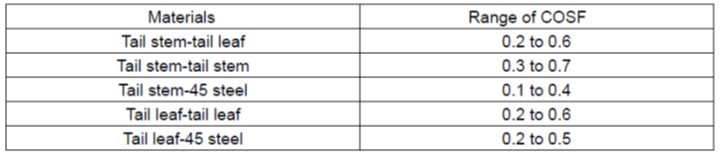The values of COSF varied greatly during the tests due to the rough surfaces of tail stem and tail leaf, and the values measured in the physical test will be used subsequently as the basis for selecting the range of parameters for the discrete element simulation test, and further calibration of COSF would be made using the stacking angle of crushed STL.

Determination of the Stacking Angle of Crushed Sugarcane Tail Leaves

The stacking angle of crushed STL was measured by the cylinder lifting test. Fresh STL were crushed according to silage length and were used as samples. Homemade steel cylinders (92 mm inner diameter and 192 mm height) were selected. The steel cylinders were filled with crushed STL samples until they were full, and the cylinders were lifted upward at a uniform speed of 0.05 m/s to form particle heap on the horizontal bottom plate, as shown in Fig. 4. The test was repeated 10 times. Grayscale processing and binarization of the stacked images of broken STL were carried out through Matlab software (MathWorks Ltd., v.2018a, Massachusetts, USA). Extraction of edge contours and linear fitting were done, and the stacking angle of crushed STL was measured as 37.52° with a COV of 4.74%.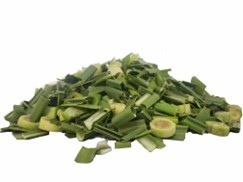Fig. 4. Particle heap of crushed STL

Simulation Model of Particle Stacking Angle

Based on the physical properties of STL measured in the previous experiment, the two parts of the crushed STL, tail stem and tail leaf, were modeled using the 3D modeling software SolidWorks (Dassault Systemes S.A, v.2018, Concord, MA, USA), and the constructed geometric models were input into engineering discrete element method (EDEM) software (DEM Solutions Ltd., v.2018, Edinburgh, UK). The material properties and contact parameters of the tail stem and tail leaf were added, and global variables were set inside the particle aggregate in the post-processing interface to provide data information for the particle factory Application Program Interface (API) compilation. Finally, the compiled two API particle factory plug-ins were added as used to build the tail stem and tail leaf discrete element model based on the Hertz Mindlin with bonding model.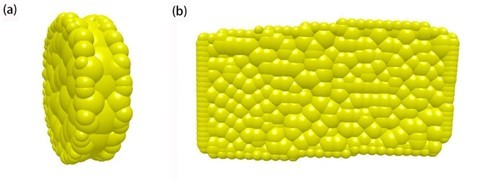Fig. 5. Discrete element model of crushed STL: (a) Tail stem; (b) Tail leaf

The spherical particles of unequal diameters were used for rapid filling to obtain the tail stem (5.6 mm in length and 18 mm in diameter) and tail leaf (2.1 cm2 in area), as shown in Fig. 5.

In the EDEM simulation software, a virtual cylinder like the steel cylinder was established as the particle factory (92 mm inner diameter and 192 mm height). To ensure the rapid and stable generation of two kinds of particles, the initial falling velocity of particles was set to 1 m/s, and the Hertz-Minglin (no slip) model was chosen for particle contact. When the generation was completed and remained stable, the cylinder was lifted upward with a speed of 0.05 m/s, and finally the particles formed a stable particle heap of crushed STL on the bottom plate, as shown in Fig. 6.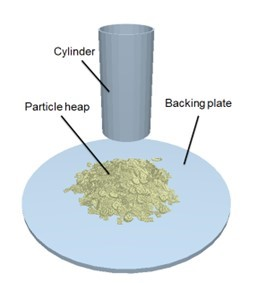Fig. 6. Stacking angle simulation model of crushed STL particles

RESULTS AND DISCUSSION

Plackett-Burman Test

The simulation test of the STL stacking angle requires many parameters. According to the values measured by the physical test, the density of tail stem and tail leaf were 987 kg/m3 and 742 kg/m3, respectively. The Poisson’s ratio and shear modulus of tail stem were 0.29 and 10.6 MPa while the Poisson’s ratio, density, and shear modulus of 45 steel were 0.30, 7850 kg/m3, and 79.4 GPa, respectively. The COSF range of the crushed STL particles was determined by the values measured in the experiment, and the simulation parameters from 14 parameters that were not easy to measure, such as Poisson’s ratio of tail leaf, shear modulus, CORF between crushed STL particles and COR between tail leaf – tail leaf or tail leaf -45 steel, were determined by reviewing the relevant literature (Wen et al. 2020). With the stacking angle of crushed STL as the response value, the Plackett-Burman test was designed using Design-Expert.11 software (Stat-Ease, Minneapolis, MN, USA) to screen out the parameter combination that has significant effects on the response value. The minimum and maximum values of the 14 uncertain parameters in Table 2 were coded as -1 and +1 levels, respectively, as shown in Table 2.

A central point was set up in the test (taking the middle value of high and low levels as the 0 level), and a total of 21 trials were conducted. The stacking angle was measured in the +X and +Y direction of crushed STL heap using the goniometric tool in the EDEM2020 software, as shown in Fig. 7, and the value was averaged. The test protocol and results are shown in Table 3.

Table 2. Plackett-Burman Test Parameters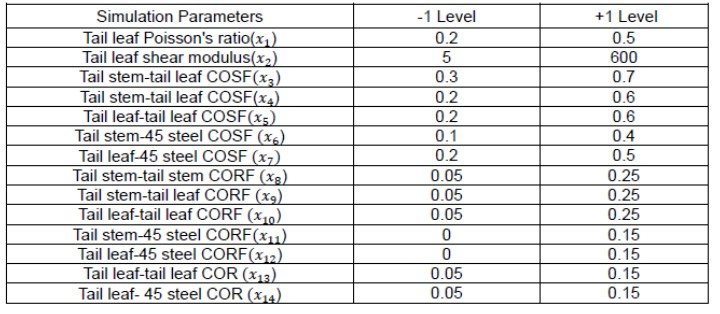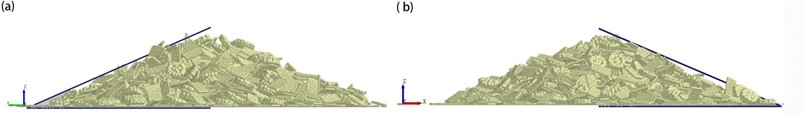Fig. 7. Discrete element model of crushed STL heap: (a) The +X direction of crushed STL heap; (b) The +Y direction of crushed STL heap

Table 3. Protocol and Result of Plackett-Burman Test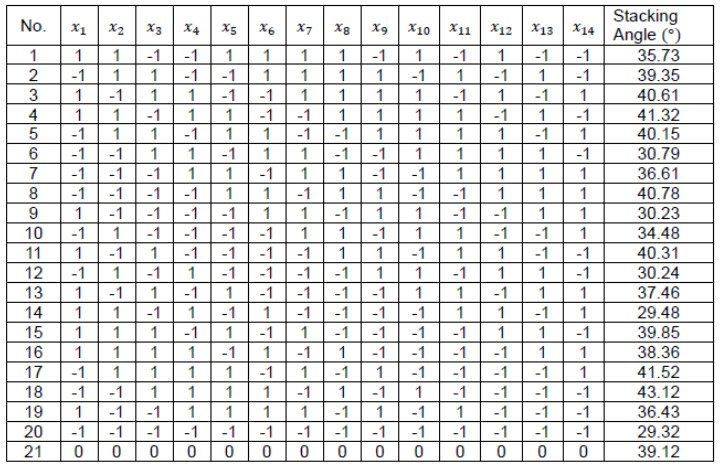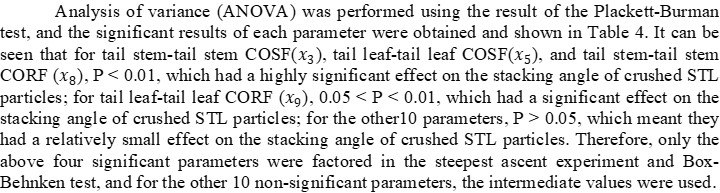Table 4. Variance and Significance Analysis of Plackett-Burman Test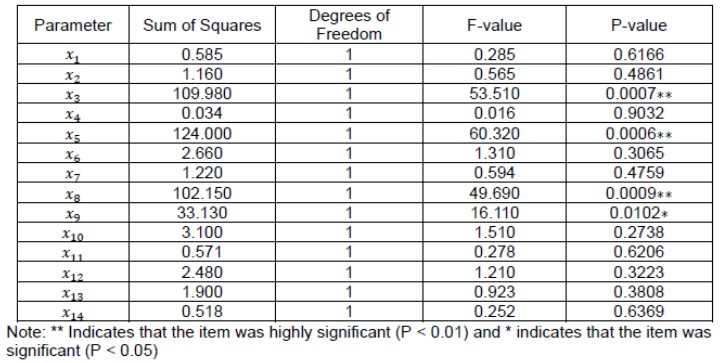Steepest Ascent Experiment

The purpose of the steepest climbing test is to determine the optimal area of significant factors, design a reasonable step size, and increase the density of the test to approach the area with the best effect. The four significant parameters obtained from the Plackett-Burman test,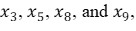were used for the steepest ascent experiment to further determine the optimal range of the significant parameters. Each significant parameter was designed to gradually increase in its range of value, and the relative errors of physical and simulated stacking angles were used as the evaluation indexes. The experimental design and results are shown in Table 5.

Table 5. Design and Result of Steepest Ascent Experiment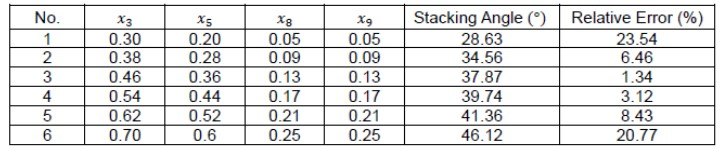The results showed that with the increasing value of the four significant parameters, the stacking angle gradually increased and the relative error showed a trend of first decreasing and then increasing. The relative error of the parameter combination corresponding to the 3rd testing group was the smallest. Therefore, the optimal parameter was near the parameter of the 3rd testing group.

Box-Behnken Test Design and Analysis of Significant Contact Parameters

According to the result of the steepest ascent experiment, the parameters of the 3rd testing group were taken as the intermediate level (0), and the parameters of the 2nd and 4th testing groups were taken as the low (-1) and high (+1) level, respectively. The values for the other 10 non-significant parameters were the same as for the steepest ascent experiment. The Box-Behnken test was designed using Design-Expert.11 software, and a total of 29 trials was performed. The test design and results are shown in Table 6.

Table 6. Box-Behnken Test Design and Result of Significant Contact Parameters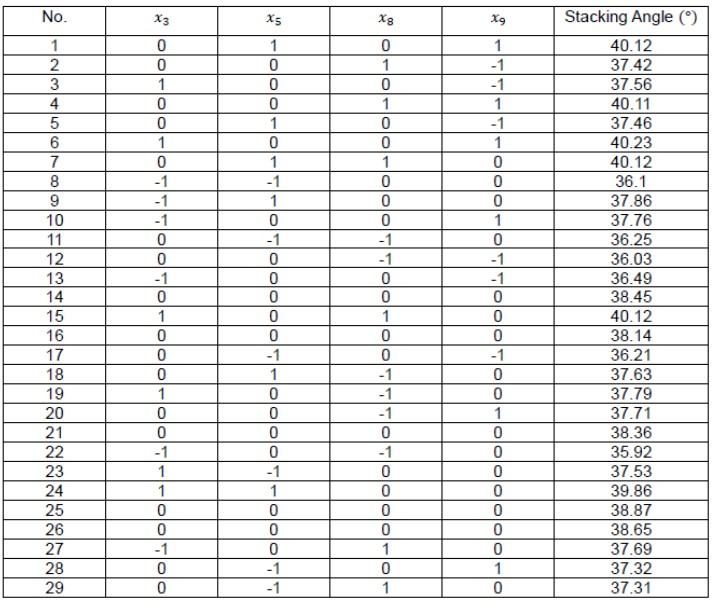Design-Expert.11 software was used for the fitting analysis of the experimental results in Table 6. The second-order regression model equation for the simulated stacking angle of crushed STL and four significant contact parameters was as follows: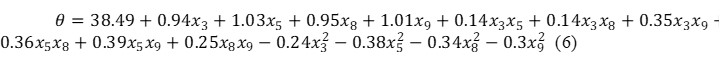The ANOVA of this regression model is shown in Table 7. For the fitting regression model P < 0.0001, the coefficient of determination was R2 = 0.989, and the corrected coefficient of determination was R2adj = 0.978. Because both were close to 1, it indicated that the regression model of stacking angle was extremely significant. The P-value of the interaction terms like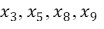and their squared terms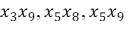was less than 0.01, and the P-value of the interaction term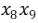was less than 0.05, indicating the significant effect of every parameter on the stacking angle. The misfitting term P = 0.9374 > 0.05 and the COV= 0.52%, indicating that the regression equation fit well and could predict the target stacking angle; the test accuracy Ap= 23.329, indicating that the model had a high accuracy.

Table 7. Variance Analysis of Box-Behnken Experimental Design Regression Model